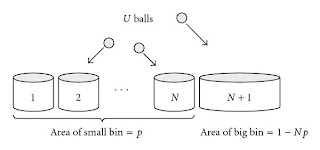## Friday, 4 September 2009

### Counting Multisets, and A New Symbol for meReading through The Endeavour, the blog of John D. Cook, I came across a new term and a symbol I had never seen, but actually quite like.

Here is a snip from what he wrote, "Suppose you have a class of 12 students. Each student will receive one of five letter grades: A, B, C, D, or F. At the end of the course, you tally up how many students received each grade. How many different ways could the tally turn out? For example, one possibility would be all A’s. Another would be three A’s, four B’s, four C’s, no D’s, and one F."

This is a special type of counting combinations, except that there can be more than one of each item. This is an example of what is called a multiset. Here is the explanation of "multiset" as it appears at Wikipedia,
"In mathematics, a multiset (or bag) is a generalization of a set. While each member of a set has only one membership, a member of a multiset can have more than one membership (meaning that there may be multiple instances of a member in a multiset, not that a single member instance may appear simultaneously in several multisets). The term "multiset" was coined by Nicolaas Govert de Bruijn in the 1970s. The use of multisets in mathematics predates the name "multiset" by nearly 90 years. Richard Dedekind used multisets in a paper published in 1888."

In fact another place on Wikipedia they mentioned that there are lots of other common names for multisets, and "Knuth also lists other names that were proposed for multisets, such as list, bunch, bag, heap, sample, weighted set, collection, and suite."

AHH, OK, I know "bag", I just never used is as a formal definition.
Ok, so how do you do a problem like that. In this case we have 5 identified things (the grades) and we want to select from this five, with replacement, until there are a total of 12. The classy way to do this uses a method called "stars and bars" and since some of my students read this, I will illustrate a little with a side note..

Suppose you are the waiter in a restaurant that sells three things, a single entree, a single type of beverage, and a dessert. You wait on a table of some number of people and each person orders one or more of the things.. Now being clever, you don't want to right down every order, so you divide your notepad into three columns by drawing two lines, remembering that the left space is for entrees, the middle one for drinks, and the last for dessert. At table two the four people ordered four entrees, two drinks and three desserts. You mark on your pad looks like xxxx | xx | xxx and when you get to the kitchen you can see what the order was..... x (stars) and | (bars) ...get it?

For 12 students and five grades we can imagine that we drew four vertical lines on a sheet of paper to separate it into five regions .... We could think of each region as representing a grade, A's to the left of the first line, B's between the first and second line, etc, with the Failing grades to the right of the fourth dividing line... the representation of three A’s, four B’s, four C’s, no D’s, and one F would then look like this; XXX | XXXX | XXXX | | X .

Note that if we identified the person who got each grade, we have created a very different problem, but for counting how many ways the 12 grades could be assigned without regard to who received each grade, we can look at the diagram and realize that if we re-order the 12 X's and four lines, we would get a different result... each order of lines and X's corresponds to exactly one assignment of 12 grades to the five letters. So the total number of ways of assigning the grades would be the same as the number of ways you could reorder the 16 characters, (lines and X's)..ie, how many ways can we pick 4 of them to be the lines???..So there are 1,820 possible grade tallies for 12 students and five grades.

Since you can stage the problem and use the notation that already exists for regular sets, it may not seem like a new symbol was necessary, but that leads, imho, to the student always trying to see the problem as a traditional combination rather than recognizing it is a different thing altogether. Perhaps that was his thinking when MIT professor Richard Stanley introduced the following symbol for the number of ways to select k things from a set of n with replacement;From John Cook, (with additions) "There are several ways to think of what this symbol represents. The first is the number of ways to select k things from a set of n with replacement. (there are five boxes labeled A,B,C,D,F and we will put students into them with replacement)
A more concrete variation on the same idea is the number of ways to place k unlabelled balls (students) into n labeled urns (grades).

So we can writeIt looks a little strange to have the bottom value larger than the top, and I admit I have never seen it written that way, but then I haven't seen it used very much... but rest assured I WILL use it in probability problems this year. And how do you read it, well just extend the idea to include the new multiset term, I would read it as "N Multi-choose K".